## Physics Highlights – May 2009June 2, 2009

Posted by dorigo in news, physics, science.
Tags: , , , , , , ,

Here is a list of noteworthy pieces I published on my new blog site in May. Those of you who have not yet updated their links to point there might benefit from it…

Four things about four generations -the three families of fermions in the Standard Model could be complemented by a fourth: a recent preprint discusses the possibility.

Fermi and Hess do not confirm a dark matter signal: a discussion of recent measurements of the electron and positron cosmic ray fluxes.

Nit-picking on the Omega_b Discovery: A discussion of the significance of the signal found by DZERO, attributed to a Omega_b particle.

Nit-picking on the Omega_b Baryon -part II: A pseudoexperiments approach to the assessment of the significance of the signal found by DZERO.

The real discovery of the Omega_b released by CDF today: Announcing the observation of the Omega_b by CDF.

CDF versus DZERO: and the winner is…: A comparison of the two “discoveries” of the Omega_b particle.

The Tevatron Higgs limits strenghtened by a new theoretical study: a discussion of a new calculation of Higgs cross sections, showing an increase in the predictions with respect to numbers used by Tevatron experiments.

Citizen Randall: a report of the giving of honorary citizenship in Padova to Lisa Randall.

Hadronic Dibosons seen -next stop: the Higgs: A report of the new observation of WW/WZ/ZZ decays where one of the bosons decays to jet pairs.

## What is the Y(4140)? The plot thickensApril 6, 2009

Posted by dorigo in news, physics, science.
Tags: , , ,

I read with interest -but it would probably be more honest to say I browsed, since I could understand less than 50%- a preprint released three days ago on “The hidden charm decay of Y(4140) by the rescattering mechanism“, by Xiang Liu, from Peking University (now at Coimbra, PT). The Y particle has been recently discovered by CDF.

The existence of the several new resonances of masses above 3 GeV recently unearthed by B factories and by the CDF experiment poses a challenge to our interpretation of these states as simple quark-antiquark bound states, because of their properties -in particular, their decay pattern and their natural widths.

Already with the first “exotic” meson discovered a few years ago (and recently measured with great precision by CDF), the X(3872), the puzzle was evident: at a mass almost coincident with twice the mass of conventional charmed mesons (states which are labeled “D”, which are composed of two quarks: a charm and a up or down quark, like$D^+ = c \bar d$ or$D^\circ = c \bar u$), the X was immediately suggested to be a molecular state of two D particles. I wrote an account of the studies of the nature of the X particle a few years ago if you are interested -but mind you, the advancements in this research field are quick, and I believe the material I wrote back then is a bit aged by now.

The paper by Liu tries to determine whether the interpretation of the Y particle as a pure second radial excitation of P-wave charmonium ($\chi_{cJ}''$, with$J=0,1$) holds water once the observed branching ratio of the Y into the final state seen by CDF ($Y \to J/\psi \phi$), and the measured decay width, are compared to a theoretical calculation.

The nice thing about the decay of the Y into the observed final state is that it occurs only through a so-called “rescattering” mechanism, by means of the diagrams shown in the graph below (the ones shown refer to the J=0 hypothesis of the$\chi_{cJ}''$, but similar diagrams are discussed for the J=1 state in the paper).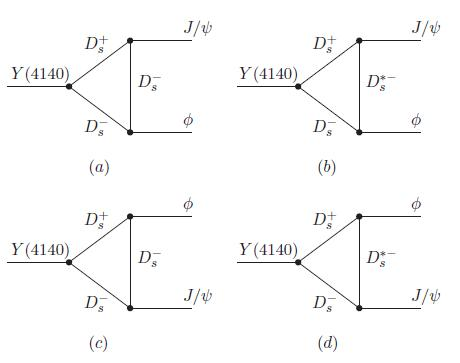As you can see, the Y produces the two final state particles by means of a triangle loop of D mesons. These diagrams usually describe rare processes, and in fact Liu’s calculations end up finding a small branching fraction. I am unable to delve into the details of the computation, so I will just state the result: the typical values of the branching ratio$B(Y \to J/\psi \phi)$ depend on a parameter,$\alpha$ which, if taken in a “reasonable” range of values, provides estimates in the ballpark of a few$10^{-4}$. This appears inconsistent with the observation provided by the CDF experiment.

Clearly, work is in progress here, so I would abstain from concluding anything definite on the matter. So, for now, let us call this an indication that the simple interpretation of the Y as a excited charmonium state is problematic.

## Streaming video for Y(4140) discoveryMarch 17, 2009

Posted by dorigo in news, physics, science.
Tags: , , , , ,

The CDF collaboration will present at a public venue (Fermilab’s Wilson Hall) its discovery of the new Y(4140) hadron, a mysterious particle created in B meson decays, and observed to decay strongly into a$J/\psi \phi$ state, a pair of vector mesons. I have described that exciting discovery in a recent post.

From this site you can connect to streaming video (starting at 4.00PM CDT, or 9.00PM GMT – should last about 1.30 hours).

## CDF discovers a new hadron!March 13, 2009

Posted by dorigo in news, physics, science.
Tags: , , , ,

This morning CDF released the results of a search for narrow resonances produced in B meson decays, and in turn decaying into a pair of vector mesons: namely,$Y \to J/\psi \phi$. This Y state is a new particle whose exact composition is as of yet unknown, except that CDF has measured its mass (4144 MeV) and established that its decay appears to be mediated by strong interactions, given that the natural width of the state is in the range of a few MeV. I describe succintly the analysis below, but first let me make a few points on the relevance of area of investigation.

Heavy meson spectroscopy appears to be a really entertaining research field these days. While all eyes are pointed at the searches for the Higgs boson and supersymmetric particles, if not at even more exotic high-mass objects, and while careers are made and unmade on those uneventful searches, it is elsewhere that action develops. Just think about it: the$\Xi_b$ baryon, the$\Omega_b$, those mysterious X and Y states which are still unknown in their quark composition. Such discoveries tell the tale of a very prolific research field: one where there is really a lot to understand.

Low-energy QCD  is still poorly known and not easily calculable. In frontier High-Energy Physics we bypassed the problem for the sake of studying high-energy phenomena by tuning our simulations such that their output well resembles the result of low-energy QCD processes in all cases where we need them -such as the details of parton fragmentation, or jet production, or transverse momentum effects in the production of massive bodies. However, we have not learnt much with our parametrizations:  those describe well what we already know, but they do not even come close to guessing whatever we do not know. Our understanding of low-energy QCD is starting to be a limiting factor in cosmological studies, such as in baryogenesis predictions. So by all means, let us pursue low-energy QCD in all the dirty corners of our produced datasets at hadron colliders!

CDF is actively pursuing this task. The outstanding spectroscopic capabilities of the detector, combined with the huge size of the dataset collected since 2002, allow searches for decays in the one-in-a-million range of branching ratios. The new discovery I am discussing today has indeed been made possible by pushing to the limit our search range.

The full decay chain which has been observed is the following:$B^+ \to Y K^+ \to J/\psi \phi K^+ \to \mu^+ \mu^- K^+ K^- K^+$. That$J/\psi$ mesons decay to muon pairs is not a surprise, as is the decay to two charged kaons of the$\phi$ vector meson. Also the original decay of the B hadron into the$J/\psi \phi K$ final state is not new: it had been in fact observed previously. What had not been realized yet, because of the insufficient statistics and mass resolution, is that the$J/\psi$ and$\phi$ mesons produced in that reaction often “resonate” at a very definite mass value, indicating that in those instances the$B \to J/\psi \phi K$ decay actually takes place in two steps as the chain of two two-body decays:$B \to Y K$ and$Y \to J/\psi \phi$.

The new analysis by CDF is a pleasure to examine, because the already excellent momentum resolution of the charged particle tracking system gets boosted when constraints are placed on the combined mass of multi-body systems. Take the B meson, reconstructed with two muons and three charged tracks, each assumed to be a kaon: if you did not know that the muon pair comes from a$J/\psi$ nor that two of the kaons come from a$\phi$, the mass resolution of the system would be in the few tens of MeV range. Instead, by forcing the momenta of the two muons to be consistent with the World average mass of the$J/\psi$,$M_{J/\psi}=3096.916 \pm 0.011 MeV$ , and by imposing that the two kaons make exactly the extremely well-known$\phi$ mass ($M_\phi=1019.455 \pm 0.020 MeV$), much of the uncertainty on the daughter particle momenta disappears, and the B meson becomes an extremely narrow signal: its mass resolution is just 5.9 MeV, a per-mille measurement event-by-event!The selection of signal events requires several cleanup cuts, including mass window cuts around the J/Psi and phi masses, a decay length of the reconstructed B+ meson longer than 500 microns, and a cut on the log-likelihood ratio fed with dE/dx and time-of-flight information capable of discriminating kaon tracks from other hadrons. After those cuts, the B+ signal really stands above the flat background. There is a total of 78+-10 events in the sample after these cuts, and this is the largest sample of such decays ever isolated. It is shown above (left), together with the corresponding distribution in the$\phi \to KK$ candidate mass (right).A Dalitz plot of the reconstructed decay candidates is shown in the figure on the right. A Dalitz plot is a scatterplot of the squared invariant mass of a subset of the particles emitted in the decay, versus the squared invariant mass of another subset. If the decay proceeds via the creation of an intermediate state, one may observe a horizontal or vertical cluster of events. Judge by yourself: do the points appear to spread evenly in the allowed phase space of the B+ decays ?

The answer is no: a significant structure is seen corresponding to a definite mass of the$J/\psi \phi$ system. A histogram of the difference between the reconstructed mass of the$J/\psi \phi$ system and the$J/\psi$ mass is shown in the plot below: a near-threshold structure appears at just above 1 GeV energy. An unbinned fit to a relativistic Breit-Wigner signal shape on top of the expected background shape shows a signal at a mass difference of$\Delta M=1046.3 \pm 2.9 MeV$, with a width of 11.7+-5.7 MeV.The significance of the signal is, after taking account of trial factors, equal to 3.8 standard deviations. For the non-zero width hypothesis, the significance is of 3.4 standard deviations, implying that the newfound structure has strong decay. The mass of the new state is thus of 4143+-2.9 MeV.

The new state is above the threshold for decay to pair of charmed hadrons. The decay of the state appears to occur to a pair of vector mesons,$J/\psi \phi$, in close similarity to a previous state found at 3930 MeV, the Y(3930), which also decays to two vector mesons in$Y \to J/\psi \omega$. Therefore, the new state can be also called a Y(4140).

Although the significance of this new signal has not reached the coveted threshold of 5 standard deviations, there are few doubts about its nature. Being a die-hard sceptic, I did doubt about the reality of the signal shown above for a while when I first saw it, but I must admit that the analysis was really done with a lot of care. Besides, CDF now has tens of thousands of fully reconstructed B meson decays available, with which it is possible to study and understand even the most insignificant nuances to every effect, including reconstruction problems, fit method, track characteristics, kinematical biases, you name it. So I am bound to congratulate with the authors of this nice new analysis, which shows once more how the CDF experiment is producing star new results not just in the high-energy frontier, but as well as in low-energy spectroscopy. Well done, CDF!

## Guess the function: resultsJanuary 21, 2009

Posted by dorigo in physics, science.
Tags: , , , , , ,

Thanks to the many offers for help received a few days ago, when I asked for hints on possible functional forms to interpolate a histogram I was finding hard to fit, I have successfully solved the problem, and can now release the results of my study.

The issue is the following one: at the LHC, Z bosons are produced by electroweak interactions, through quark-antiquark annihilation. The colliding quarks have a variable energy, determined by probability density functions (PDF) which determine how much of the proton’s energy they carry; and the Z boson has a resonance shape which has a sizable width: 2.5 GeV, for a 91 GeV mass. The varying energy of the center of mass, determined by the random value of quark energies due to the PDF, “samples” the resonance curve, creating a distortion in the mass distribution of the produced Z bosons.

The above is not the end of the story, but just the beginning: in fact, there are electromagnetic corrections (QED) due to the radiation of photons, both “internally” and by the two muons into which the Z decays (I am focusing on that final state of Z production: a pair of high-momentum muons from$Z \to \mu^+ \mu^-$). Also, electromagnetic interactions cause a interference with Z production, because a virtual photon may produce the same final state (two muons) by means of the so-called “Drell-Yan” process. All these effects can only be accounted for by detailed Monte Carlo simulations.

Now, let us treat all of that as a black box: we only care to describe the mass distribution of muon pairs from Z production at the LHC, and we have a pretty good simulation program, Horace (developed by four physicists in Pavia University: C.M. Carloni Calame, G. Montagna, O. Nicrosini and A. Vicini), which handles the effects discussed above. My problem is to describe with a simple function the produced Z boson lineshape (the mass distribution) in different bins of Z rapidity. Rapidity is a quantity connected to the momentum of the particle along the beam direction: since the colliding quarks have variable energies, the Z may have a high boost along that direction. And crucially, depending on Z rapidity, the lineshape varies.

In the post I published here a few days ago I presented the residual of lineshape fits which used the original resonance form, neglecting all PDF and QED effects. By fitting those residuals with a proper parametrized function, I was trying to arrive at a better parametrization of the full lineshape.

After many attempts, I can now release the results. The template for residuals is shown below, interpolated with the function I obtained from an advice by Lubos Motl: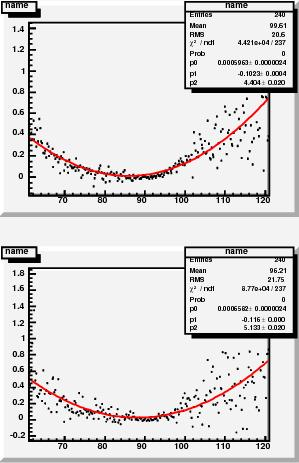After multiplying that function by the original Breit-Wigner resonance function, I could fit the 24 lineshapes extracted from a binning in rapidity. This produced additional residuals, which are of course much smaller than the first-order ones above, and have a sort of parabolic shape this time. A couple of them are shown on the right.

I then interpolated those residuals with parabolas, and extracted their fit parameters. Then, I could parametrize the parameters, as the graph below shows: the three degrees of freedom have roughly linear variations with Z rapidity. The graphs show the five parameter dependences on Z rapidity (left column) for lineshapes extracted with the CTEQ set of parton PDF; for MRST set (center column); and the ratio of the two parametrization (right column), which is not too different from 1.0.Finally, the 24 fits which use the$f(m,y)$ shape, with now all of the rapidity-dependent parameters fixed, are shown below (the graph shows only one fit, click to enlarge and see all of them together).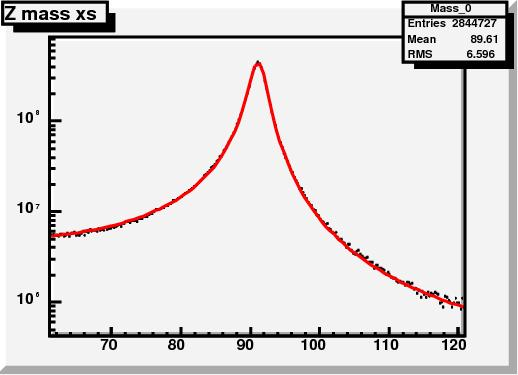The function used is detailed in the slide below:I am rather satisfied by the result, because the residuals of these final fits are really small, as shown on the right: they are certainly smaller than the uncertainties due to PDF and QED effects. The$f(m,y)$ function above will now be used to derive a parametrization of the probability that we observe a dimuon pair with a given mass$m$ at a rapidity$y$, as a function of the momentum scale in the tracker and the muon momentum resolution.

## An exam in Subnuclear PhysicsJanuary 9, 2009

Posted by dorigo in physics.
Tags: , , , , , , , ,

Here are the questions asked at an exam in Subnuclear Physics this morning:

• Draw the strong and electromagnetic coupling constants as a function of$Q^2$, explain their functional dependence using feynman graphs of the corrections to the photon and gluon propagators, write their formula, and compute the value of the constants at$Q^2=M_Z^2$, given the values at$Q^2=1 MeV^2$ (QED) and$Q^2=1 GeV^2$ (QCD).
• The GIM mechanism: explain the need for a fourth quark using box diagrams of kaon decays to muon pairs. How does the charm contribution depend on its mass ? What conclusion could be drawn by that dependence in the case of B mixing measurements in the eighties ?
• Discuss a measurement of the top quark mass. For a dileptonic decay of top quark pairs, discuss the final state and its production rate.
• Discuss decay modes of W bosons and their branching fraction values. Discuss decay modes of Z bosons and their branching fraction values.

The student answered well all questions and he got 30/30 points.

## Some posts you might have missed in 2008 – part IIJanuary 6, 2009

Posted by dorigo in physics, science.
Tags: , , , , , , , , , , , ,

Here is the second part of the list of useful physics posts I published on this site in 2008. As noted yesterday when I published the list for the first six months of 2008, this list does not include guest posts nor conference reports, which may be valuable but belong to a different place (and are linked from permanent pages above). In reverse chronological order:

December 29: a report on the first measurement of exclusive production of charmonium states in hadron-hadron collisions, by CDF.

December 19: a detailed description of the effects of parton distribution functions on the production of Z bosons at the LHC, and how these effects determine the observed mass of the produced Z bosons. On the same topic, there is a maybe simpler post from November 25th.

December 8: description of a new technique to measure the top quark mass in dileptonic decays by CDF.

November 28: a report on the measurement of extremely rare decays of B hadrons, and their implications.

November 19, November 20, November 20 again , November 21, and November 21 again: a five-post saga on the disagreement between Lubos Motl and yours truly on a detail on the multi-muon analysis by CDF, which becomes a endless diatriba since Lubos won’t listen to my attempts at making his brain work, and insists on his mistake. This leads to a back-and-forth between our blogs and a surprising happy ending when Motl finally apologizes for his mistake. Stuff for expert lubologists, but I could not help adding the above links to this summary. Beware, most of the fun is in the comments threads!

November 8, November 8 again, and November 12: a three-part discussion of the details in the surprising new measurement of anomalous multi-muon production published by CDF (whose summary is here). Warning: I intend to continue this series as I find the time, to complete the detailed description of this potentially groundbreaking study.

October 24: the analysis by which D0 extracts evidence for diboson production using the dilepton plus dijet final state, a difficult, background-ridden signature. The same search, performed by CDF, is reported in detail in a post published on October 13.

September 23: a description of an automated global search for new physics in CDF data, and its intriguing results.

September 19: the discovery of the$\Omega_b$ baryon, an important find by the D0 experiment.

August 27: a report on the D0 measurement of the polarization of Upsilon mesons -states made up by a$b \bar b$ pair- and its relevance for our understanding of QCD.

August 21: a detailed discussion of the ingredients necessary to measure with the utmost precision the mass of the W boson at the Tevatron.

August 8: the new CDF measurement of the lifetime of the$\Lambda_b$ baryon, which had previously been in disagreement with theory.

August 7: a discussion of the new cross-section limits on Higgs boson production, and the first exclusion of the 170 GeV mass, by the two Tevatron experiments.

July 18: a search for narrow resonances decaying to muon pairs in CDF data excludes the tentative signal seen by CDF in Run I.

July 10: An important measurement by CDF on the correlated production of pairs of b-quark jets. This measurement is a cornerstone of the observation of anomalous multi-muon events that CDF published at the end of October 2008 (see above).

July 8: a report of a new technique to measure the top quark mass which is very important for the LHC, and the results obtained on CDF data. For a similar technique of relevance to LHC, also check this other CDF measurement.

## What is a glueball ?January 4, 2009

Posted by dorigo in Blogroll, physics, science.
Tags: , , ,

This post is just a placeholder for a link and an invitation for you to join me and ask Marco Frasca to further his already enlightening discussion of glueballs, as I already did in the comments thread of his post.

The subject is indeed fascinating: gluonic matter. A condensate of bosons. Asymptotic states (particles) made of no fermions. Matter as we never experienced it.

Glueballs are (thought to be) bound states of gluons. The gluon, the carrier of strong interactions, is a massless boson, and it is charged with the attribute it mediates: colour. Because of the colour charge of gluons, these particles can interact with one another, giving rise to the fascinating properties of Quantum Chromodynamics, the asymptotic freedom properties of bound states of quarks, and the infrared slavery – the impossibility of obtaining free coloured objects (quarks or gluons). The non-abelian nature of the SU(3) group underlying quantum chromodynamics is a source of the difficulties of calculating the low-energy limit of the theory.

The self-interaction properties of gluons creates the possibility of a color-neutral state made of gluons only: glueballs. Glueball properties, however, cannot be computed with perturbation theory, and these remain very mysterious objects over thirty years after QCD was understood in its workings. Only with lattice calculations -you know, discretizing space and computing path integrals with finite sums- can the glueballs be understood. But Marco hints at methods that allow a deeper understanding: methods based on condensed matter physics. We are all ears!

## Three exquisite exclusive charmonium signalsDecember 29, 2008

Posted by dorigo in news, physics, science.
Tags: , , ,

It is my pleasure today to report on an extremely interesting measurement recently produced by the CDF experiment, one which is dear to me for several reasons.

First of all, the measurement involves the study of charmonium -a fascinating system made by a charm-anticharm quark pair, the two quarks orbiting around each other for a tiny fraction of a second before annihilating. Charmonium -in the form of the$J/\psi$ particle- is the state of matter discovered in November 1974 by Nobel Laureates Samuel Ting (at Brookhaven) and Burton Richter (at SLAC), and which immediately confirmed the reality of quarks -entities whose reality had kept many brilliant minds skeptical during the previous decade. The$J/\psi$ and the other charmonium states have been since then a gold mine for the study of Quantum Chromodynamics, of B hadron phenomenology (through the easy-to-spot decays of the type$B \to J/\psi X$), and an invaluable calibration tool for particle trackers. Charmonium may one day be even used with profit to measure with high precision the top-quark mass at the LHC!

Second, the study focuses on what is called “exclusive production”, a spectacular signature at hadron colliders: it involves the production of the particle without the disintegration of the projectiles, through a colourless exchange. Basically, the charmonium meson appears out of nothing, and in the company of exactly nothing else. It is as if by a stroke of a magic wand, a spark is exchanged between a proton and an antiproton coming close together, and a single particle is created. Those of you who know what kind of a mess is routinely yielded by proton-antiproton collisions at 2 TeV of energy in the core of CDF and D0 cannot but join my awe for the very concept of clean single-body production.

Third, exclusive production processes are quite rare in hadron collisions, and they are extremely interesting because they may, one day, provide the means by which we can access a direct measurement of the quantum numbers of the Higgs boson at the LHC. Also, their theoretical calculation is not on very solid ground, so a measurement is of great help. This one by CDF is the first ever on exclusive charmonium in hadron-hadron collisions.

Some notes on the production mechanisms

Before I come to describe the measurement details, let me present you with two Feynman diagrams of the simplest reactions responsible for the exclusive creation of a charmonium system. As I said above, the exchange between proton and antiproton is colourless: this, while still being a QCD interaction! But how is it possible for quantum chromodynamics to mediate a colourless reaction ? We know that the carrier of the strong force is the gluon, a bi-coloured object. On the other hand, if a proton emits or absorbs a gluon, it will never keep intact: it will become coloured, and explode like a firework. Unless…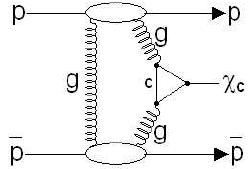Unless a second gluon immediately comes to the rescue, restoring the neutrality of the proton! This is what is needed for a strong interaction to produce a charmonium state: two gluons are emitted and absorbed by the proton and the antiproton, and the pair can thus leave the interaction point, slightly rattled but unharmed. The first pair of gluons meets halfways, creating a charm-quark loop. The loop then “closes” into a$\chi_c$ meson, a charmonium state with the right quantum numbers. This is the process shown on the right.

Notice that a two-gluon exchange cannot give rise to a$J/\psi$ meson. This is because of the invariance of strong interactions for the operation called “charge conjugation”. A discussion of the invariance properties of particle reactions under the operation of exchanging every particle with its antiparticle would take us too far, and is the proper subject of another post. I will just say here that the reaction is impossible: in quantum mechanics, one says that the “transition amplitude vanishes” for a two-gluon state converting into a vector particle.

Not so for the$\chi_c$ though, since that particle has zero angular momentum: it is not a vector, but a pseudoscalar meson. That is why we have examined$\chi_c$ production first. But exclusive$J/\psi$ production does occur anyway.

How, however, if we are to keep the two incoming baryons intact? Let us think at the problem from the point of view of the$J/\psi$ then. This particle is known to be quite slow to decay into hadrons. In fact, it has a lifetime much longer than similar hadronic states. So much so, in fact, that the very suppressed electromagnetic decay into electron or muon pairs gets a sizable share of the probability. An electromagnetic process competing with a strong process ? This is very weird: we know that the electromagnetic decay must have a rate proportional to the coupling constant$\alpha$, which is fifty times smaller than the strong coupling constant$\alpha_s$ for such low-energy phenomena. However, how is quantum chromodynamics going to disintegrate a$J/\psi$ ?

Phase one is simple: the charm and the anticharm get together in the same point of space, annihilating. Phase two is the emission of gluons: not a single one though, because the$J/\psi$ is colourless! And not just two, because of the quantum mechanical selection rule concerning C-parity we mentioned above! The minimum number of gluons is three. That means that we have to take the third power of$\alpha_s$ when computing the decay rate through QCD interactions, and so electromagnetic processes become competitive.

In reality, these three-gluon exchanges are even more suppressed than they ought to be. This is encoded in what is called “OZI rule”, by the name of Okubo, Zweig, and Iizuka: at any order of the strong coupling constant$\alpha_s$, “disconnected” strong interaction processes -ones that have no fermion lines propagating from the initial to the final state, but only gluons- occur with smaller frequency. This is a consequence of the variability of$\alpha_s$ with the energy of the gluon emission: in disconnected diagrams gluons have to carry an energy at least equal to the mass of the particles they are going to materialize in the final state, and the corresponding value of the coupling constant is therefore evaluated at a higher energy, and is thus much smaller than the one involved in the emission of softer gluons in non-disconnected diagrams. Confused ? Not a problem, forget the argument and let us return to$J/\psi$ production.

The above discussion just meant to show that we need three gluons to produce one$J/\psi$, since we now know that one such particle can decay to no less than three gluons. Now, let us take the protons -the projectiles producing the reaction- in the equation. Who is going to provide two gluons, and who is going to provide only one ? I can picture the proton and antiproton starting a bitter argument on this issue. The one who provides a single gluon is a dead baryon, since it will get its colourlessness unbalanced, and it will disgregate. This is bad, because the production process is not exclusive any longer if, together with a$J/\psi$ meson, we get additional hadrons.How then can we produce a vector charmonium particle in an exclusive fashion ? Electrodynamics comes to the rescue. One of the two projectiles yields a pair of gluons, and the other emits a photon. Both can remain intact this way, and angular momentum does not object. The process is shown on the right: as you see, the charm-anticharm pair becomes a colourless one after the second gluon is exchanged, and a$J/\psi$ (or a$\psi(2S)$ meson, which has the same properties except angular momentum) arises.

The CDF search

Now that we know how proton-antiproton collisions are expected to yield exclusive charmonium, we may look for it more meaningfully.

The search uses an integrated luminosity of 1.5 inverse femtobarns of proton-antiproton collisions, recorded by the CDF experiment during Run II. The trigger used to collect exclusive production candidates selects data with two muon tracks of opposite charge, with no energy recorded in the BSC1 system, a set of scintillation counters covering the rapidity intervals$5.4 < |\eta| < 5.9$. Such a requirement excludes data which may be similar kinematically to real exclusive production, but feature a few hadrons produced almost parallel to the proton-antiproton direction.

Offline, events are excluded if they contain any other detected particle but the two muons. However, the search for$\chi_c$ allows one electromagnetic energy cluster in the calorimeter, signalling the potential photon emitted along with two muons in the$\chi_c \to \mu^+ \mu^- \gamma$ decay.

After those cuts, the only potential background to address is cosmic-ray muons that cross the detector, leaving a long track that may be fit as a pair of opposite-charge muons if it crosses the detector in proximity of the beam. These events are rejected by requiring that the two muons have an azimuthal opening angle smaller than 3.0 radians, and rejecting ones that have timing information incompatible with the instant when the proton and antiproton bunches cross in the detector.

The invariant mass of the 402 events surviving the selection is histogrammed in the graph below, if it is in the 3.0 – 4.0 GeV range (ignore the inset, which shows a fit to the background). A clear peak of$J/\psi$ and$\psi(2S)$ candidates is apparent on a flat background of non-resonant QED dimuon pairs; the set of events in the$J/\psi$ peak which contain one additional photon candidate make the$\chi_c$ signal (65 events).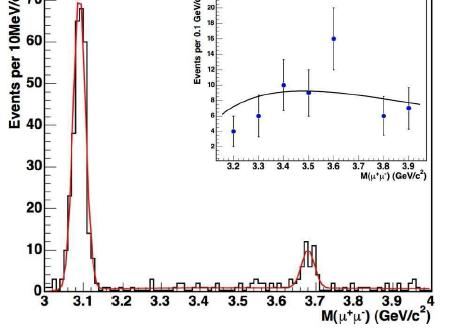The calculation of efficiencies is complicated by the fact that the exclusivity requirements remove bunch crossings which produced more than a single proton-antiproton interaction; this is a function of the number of particles in the bunches. After all is accounted properly, CDF can however determine the cross-section for charmonium production in exclusive processes with a good accuracy. The results are as follows:

•$\frac{d \sigma}{dy} (y=0) = 3.92 \pm 0.62 nb$ for$J/\psi$
•$\frac {d \sigma}{dy} (y=0) = 0.54 \pm 0.15 nb$ for$\psi(2S)$
•$\frac {d \sigma}{dy} (y=0) = 75 \pm 14 nb$ for$\chi_c$

The above results are in agreement with theoretical calculations, which have very large uncertainties. This measurement was long overdue: it is a very important input for theoretical calculations of exclusive processes.

The kinematical characteristics of the resulting$J/\psi$ and$\psi(2S)$ decay signals are studied by comparing data with a Monte Carlo simulation of photoproduction, finding good agreement. Below are shown the transverse momentum distributions of the resonances in the data (red points), compared to simulation (black histograms).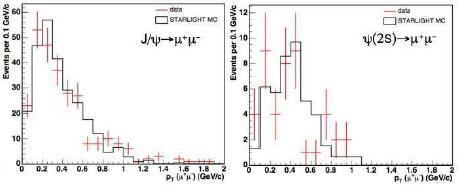In conclusion, I am happy to see these very intriguing signals appear in CDF data, and I congratulate with the main authors of the analysis, Long Zhang, Mike Albrow, and James Pinfold! You can find more information on the analysis in the public web page of the result.

## What has been going on aroundDecember 14, 2008

Posted by dorigo in Blogroll, internet.
Tags: , , , , ,